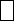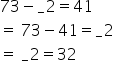Mathematics
Easy

Question

# Find the missing digit in the equation.73 -2 = 41

## 5432Hint:

## The correct answer is: 3

###73 – 41 = 32The missing digit in the equation is 3

This is a basic linear equation

### Related Questions to study#### With Turito Foundation.#### Get an Expert Advice From Turito.